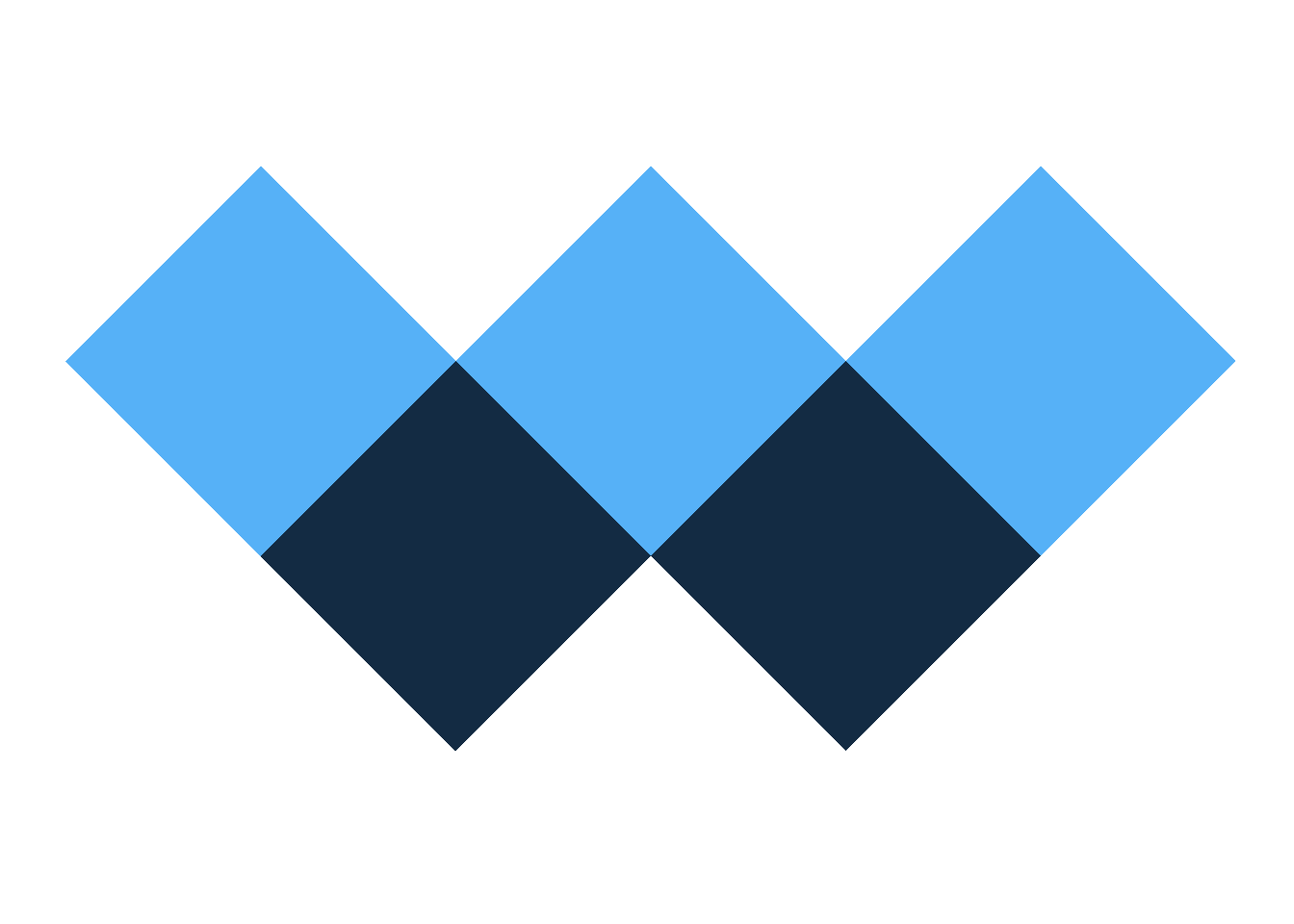# Generate My Personal Logo

I generated my personal logo with the following code. It was fun.``library(tidyverse)``

The squares are represented by `x,y` coordinates, together with `id` and `color`:

``````gap <- 0.0
square <- tibble(y = c(0,1,2, 1), x = c(0,1,0,-1))
square2 <- mutate(square, x = x - 1 - gap, id = 2, color = 1)
square4 <- mutate(square, x = x + 1 + gap, id = 4, color = 1)
square3 <- mutate(square, y = y + 1 + gap, id = 3, color = 2)
square1 <- mutate(square, x = x - 2 - 2*gap, y = y + 1 + gap, id = 1, color = 2)
square5 <- mutate(square, x = x + 2 + 2*gap, y = y + 1 + gap, id = 5, color = 2)
squares <- bind_rows(square1, square2, square3, square4, square5)``````

Logo is rendered through `geom_polygon()`:

``````ggplot(data = squares) +
geom_polygon(aes(x = x, y = y, group = id, fill = color), show.legend = F) +
coord_fixed() +
theme_void()``````